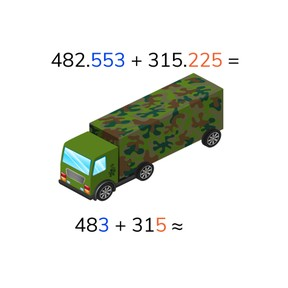Estimated and exact sum with decimal numbers with 3 decimal places

# Estimated and exact sum with decimal numbers with 3 decimal places

No account needed.8,000 schools use Gynzy92,000 teachers use Gynzy1,600,000 students use Gynzy

## General

Students learn to add decimal numbers with up to 3 decimal places and to estimate the sum and calculate the exact sum.

## Common core standard(s)

CCSS.Math.Content.6.NS.B.3

## Relevance

It is useful to be able to calculate the estimated and exact sum of decimal numbers with 3 decimal places, so you can use the estimated total as a check to your exact sum. You need this to determine about how much objects weigh together, for example.

## Introduction

The interactive whiteboard shows decimal numbers with 1, 2, and 3 decimal points. Students must determine their order and set them in order from least to greatest. You can drag the numbers to the circles on the interactive whiteboard. Emphasize that a decimal number with 3 decimal places is not always greater than a decimal number with 1 or 2 decimal places.

## Development

Check that students are able to calculate the estimated and exact sum of decimal numbers with 3 decimal places by asking the following questions:
- How do you round decimal numbers to determine the estimated sum?
- How do you add decimal numbers if you want to determine the exact sum?
- What is useful to do when adding decimal numbers with 1, 2 and 3 decimal places?

## Guided practice

Students first practice adding decimal numbers with 2 and 3 decimal places. Then they are asked to solve using a story problem.

## Closing

Discuss again why it is important to be able to calculate the estimated and exact sum of decimal numbers with 3 decimal places. By using the estimated sum to act as a check of your exact sum, you addition becomes quicker, easier and more accurate. Check that students are able to add the decimal numbers by splitting the second addend or by splitting both addends by doing the exercises on the interactive whiteboard as a class. Finally students must determine which child has thrown their spear the furthest. Ask how they determined their answer.

## Teaching tips

Students who have difficulty with this can be supported by adding on a number line. Encourage them to take smaller jumps in adding so they understand the progression.

### The online teaching platform for interactive whiteboards and displays in schools

• Save time building lessons

• Manage the classroom more efficiently

• Increase student engagement